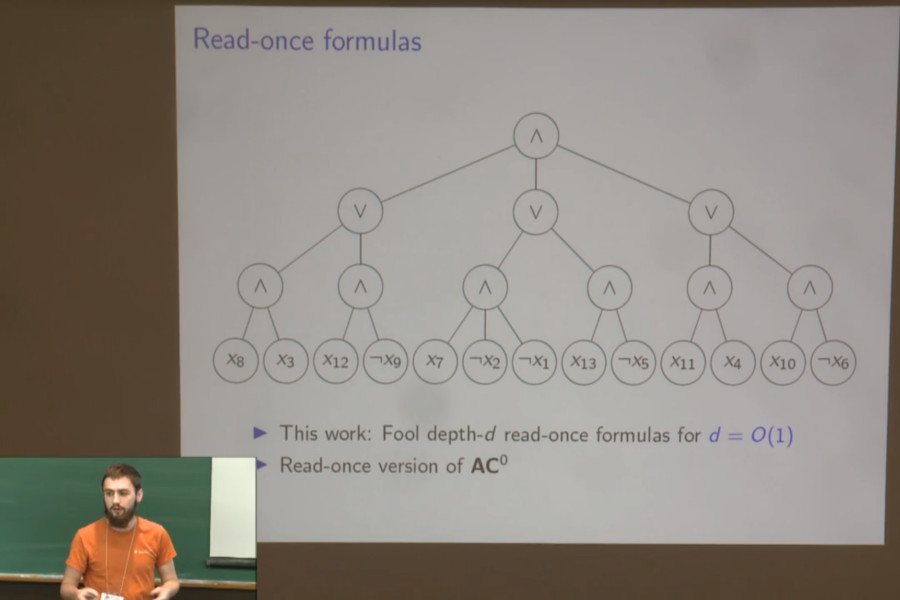Back to list of papers

# Near-Optimal Pseudorandom Generators for Constant-Depth Read-Once Formulas

By Dean Doron, Pooya Hatami, and William M. Hoza

Abstract (for specialists)

We give an explicit pseudorandom generator (PRG) for read-once $$\mathbf{AC}^0$$, i.e., constant-depth read-once formulas over the basis $$\{\wedge, \vee, \neg\}$$ with unbounded fan-in. The seed length of our PRG is $$\widetilde{O}(\log(n/\varepsilon))$$. Previously, PRGs with near-optimal seed length were known only for the depth-2 case (Gopalan et al. FOCS '12). For a constant depth $$d > 2$$, the best prior PRG is a recent construction by Forbes and Kelley with seed length $$\widetilde{O}(\log^2 n + \log n \log(1/\varepsilon))$$ for the more general model of constant-width read-once branching programs with arbitrary variable order (FOCS '18). Looking beyond read-once $$\mathbf{AC}^0$$, we also show that our PRG fools read-once $$\mathbf{AC}^0[\oplus]$$ with seed length $$\widetilde{O}(t + \log(n/\varepsilon))$$, where $$t$$ is the number of parity gates in the formula.

Our construction follows Ajtai and Wigderson's approach of iterated pseudorandom restrictions (Advances in Computing Research '89). We assume by recursion that we already have a PRG for depth-$$d$$ $$\mathbf{AC}^0$$ formulas. To fool depth-$$(d + 1)$$ $$\mathbf{AC}^0$$ formulas, we use the given PRG, combined with a small-bias distribution and almost $$k$$-wise independence, to sample a pseudorandom restriction. The analysis of Forbes and Kelley shows that our restriction approximately preserves the expectation of the formula. The crux of our work is showing that after $$\text{poly}(\log \log n)$$ independent applications of our pseudorandom restriction, the formula simplifies in the sense that every gate other than the output has only $$\text{polylog } n$$ remaining children. Finally, as the last step, we use a recent PRG by Meka, Reingold, and Tal (STOC '19) to fool this simpler formula.

Not-so-abstract (for curious outsiders)

⚠️ This summary might gloss over some important details.

A "pseudorandom generator" is an algorithm that makes a few coin tosses and outputs a long sequence of bits $$(x_1, x_2, x_3, \dots)$$ that "look random" in some sense. In this paper, we present a pseudorandom generator whose output looks random in the following sense. Consider a statement that says something like $((x_3 = 0 \text{ AND } x_7 = 1 \text{ AND } x_2 = 1) \text{ OR } (x_6 = 1 \text{ AND } (x_5 = 0 \text{ OR } x_1 = 0) \text{ AND } \dots)).$ Each variable is only allowed to appear one time, and the parentheses are only allowed to be nested to a depth of, say, 100. For every statement of that form, the probability that the statement evaluates to true when you plug in the output of our pseudorandom generator is roughly the same as the probability that the statement evaluates to true when you plug in truly random bits. Our pseudorandom generator is "near-optimal", i.e., our algorithm makes very few coin tosses.

We posted a manuscript online in November 2018; I presented the paper at CCC in July 2019. The CCC proceedings version has some minor presentational improvements compared to the ECCC version.

Expository material:Video from my presentation at BIRS workshop 19w5088 (July 2019). Here are the slides from that presentation; I used very similar slides for my presentation for my "Research Preparation Exam" (May 2019), part of the UT CS PhD program. See also these shorter slides from my presentation at CCC (July 2019).

🎓

An exposition of the paper is included in my PhD dissertation (Section 5.2).

☢️ Errata:

• (October 2021) The proof of Lemma 14 is not quite correct. To ensure that we can use the bound $${M \choose k} \geq (M/k)^k$$, we should replace $$M$$ with $$\max\{M, k\}$$. The result is that in the conclusion of the lemma, instead of the bound $$\Delta(\phi|_X) \leq 10\sqrt{\Delta(\phi)} \log^2(1/\theta)$$, we should have the bound $$\Delta(\phi|_X) \leq O(\sqrt{\Delta(\phi)} \log^2(1/\theta) + \log(1/\epsilon_0))$$. The main results of the paper are unaffected.